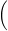For a person exercising, the velocity v (in liters per second) of airflow during a respiratory cycle (the time from the beginning of one breath to the beginning of the next) is modeled by

v = 1.8 sin (3.14t/5)where t is the time (in seconds). (Inhalation occurs when v > 0, and exhalation occurs when v < 0.)Time in seconds is along the horizontal axis and the air velocity is the vertical axis. A full cycle is 10 seconds, so the frequency is 60/10=6 times a minute, or 6 cycles a minute. The sine is zero when t=5 seconds, because we then have sin(π) (3.14 represents pi). The graph shows inhalation as the red curve above the axis and exhalation below the axis. (You can also see that the maximum airflow velocity magnitude 1.8L/s is at 2.5 seconds (inhalation) and 7.5 seconds (exhalation) and every 5 seconds thereafter.)

by Top Rated User (721k points)